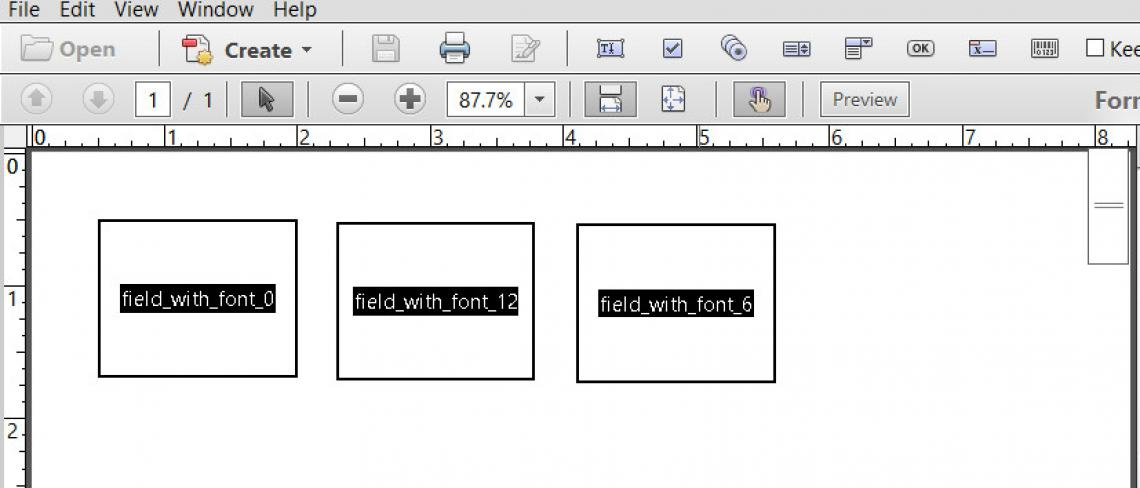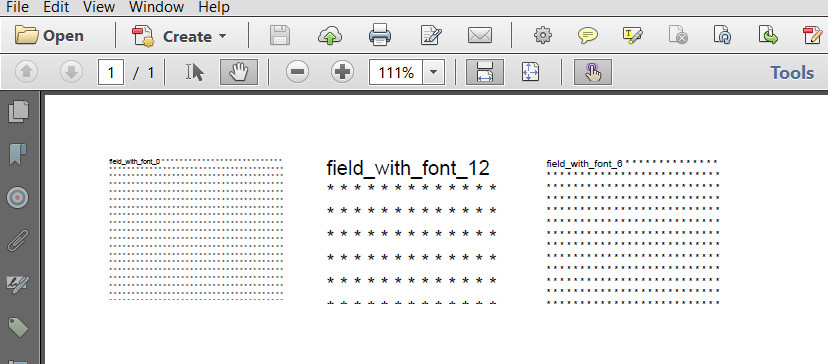iText pdf library

# How to get the row count of a multiline field?

I can add text using iText, but don't know how to calculate the right amount of filler to add.

I have a PDF with AcroFields. Some fields are multi-line fields. I have a requirement to populate AcroFields with given text and pad any remaining space in the field with an "*" (or a predefined character/string). I can add text using iText, but don't know how to calculate the right amount of filler to add.

Posted on StackOverflow on Nov 19, 2015 by Chamila

Consider a form with three multi-line text fields:Form with three multiline fields

For the first field, we defined a font size 0 (which is also what you do); for the second field, we define a font 12; for the third field, we define a font 6.

Now let's fill out and flatten the form:

``````public void manipulatePdf(String src, String dest) throws DocumentException, IOException {
PdfStamper stamper = new PdfStamper(reader, new FileOutputStream(dest));
AcroFields form = stamper.getAcroFields();
StringBuilder sb = new StringBuilder();
for (String name : form.getFields().keySet()) {
int n = getInformation(form, name);
for (int i = 0; i ``````

The result looks like this:Filled out multiline fields

As you can see, the number of `*` we add as filler depends on the font size that was defined at the field level. If the font size is 0, the font will be adapted in such a way that the text always fits. In case the font has an actual value, we can more or less calculate the number of lines and "columns" we'll need:

``````public int getInformation(AcroFields form, String name) {
form.getFieldItem(name);
AcroFields.Item item = form.getFieldItem(name);
PdfDictionary dict = item.getMerged(0);
PdfString da = dict.getAsString(PdfName.DA);
Object[] da_values = AcroFields.splitDAelements(da.toUnicodeString());
if (da_values == null) {
System.out.println("No default appearance");
}
BaseFont bf = null;
String font = (String)da_values[AcroFields.DA_FONT];
if (font != null) {
PdfDictionary dr = dict.getAsDict(PdfName.DR);
if (dr != null) {
PdfDictionary fontDict = dr.getAsDict(PdfName.FONT);
bf = BaseFont.createFont((PRIndirectReference)fontDict.get(new PdfName(font)));
}
}
if (bf == null) {
System.out.println("No BaseFont");
}
else {
System.out.println("Basefont: " + bf.getPostscriptFontName());
System.out.println("Size: " + da_values[AcroFields.DA_SIZE]);
Float size = (Float)da_values[AcroFields.DA_SIZE];
if (size == 0)
return 1000;
Rectangle rect = form.getFieldPositions(name).get(0).position;
float factor = bf.getFontDescriptor(BaseFont.BBOXURY, 1) - bf.getFontDescriptor(BaseFont.BBOXLLY, 1);
int rows = Math.round(rect.getHeight() / (size * factor) + 0.5f);
int columns = Math.round(rect.getWidth() / bf.getWidthPoint(" *", size) + 0.5f);
System.out.println("height: " + rect.getHeight() + "; width: " + rect.getWidth());
System.out.println("rows: " + rows + "; columns: " + columns);
return rows * columns;
}
return 1000;
}
``````

First we get the font so that we can create a `BaseFont` object. Then we get the font size and using information stored in the `BaseFont`, we'll define the factor that will be used to calculate the leading (that's the space between two lines).

We also ask the field for its dimensions. We then calculate how many lines we can fit into the height (`rows`) and how many times we can fit the `String " *"` into the width (`columns`) of the field's rectangle. If we multiply `columns` and `rows`, we get an approximate value of how many times we have to add `" *"` to get the appropriate filler. It doesn't matter if we have too much filler: if a font is defined, all the text that doesn't fit will be dropped.

You can find the full example here: MultiLineFieldCount

We take the form multiline.pdf and the `getInformation()` method returns this information:

``````Basefont: Helvetica
Size: 0.0
Basefont: Helvetica
Size: 6.0
height: 86.0; width: 108.0
rows: 13; columns: 27
Basefont: Helvetica
Size: 12.0
height: 86.0; width: 107.999985
rows: 7; columns: 14
``````

We can't tell much about the first field, because the font size is 0. That is also the case in your example. If the font is 0, your question is unanswerable. There is just no way you can calculate how many `*` fit into the field, because you don't know the font size that will be used to render `*`.

If the font size is 12, we can fit 7 rows and 14 colums (we see 7 rows and 13 columns in the screen shot). If the font size is 6, we can fit 14 rows and 27 columns (we see 14 rows and 26 columns in the screen shot).

The extra column is caused by the fact that we used the `ceil()` method. It's better to overestimate the number of columns than to underestimate it...

Contact

## Still have questions?

We're happy to answer your questions. Reach out to us and we'll get back to you shortly.